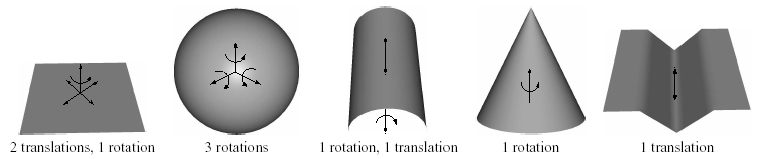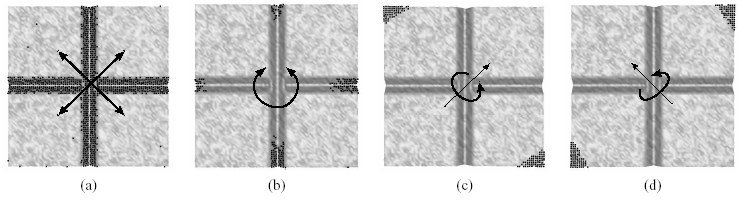# Geometrically Stable Sampling for the ICP Algorithm

 Natasha Gelfand Stanford University Leslie Ikemoto Stanford University Szymon Rusinkiewicz Princeton University Marc Levoy Stanford University

Fourth International Conference on
3D Digital Imaging and Modeling
(3DIM 2003)
October 6-10, 2003By analyzing the eigenvalues and eigenvectors of the covariance matrix derived from the points on the shapes, we can determine that the above shapes are unstable. Below each figure is the number and type of instabilities. The axis of unstable rotation and direction of unstable translation are indicated on the shapes.Points picked by our algorithm to constrain the unstable motions of a patch with two grooves. (a) Points constraining two unstable translational eigenvectors. (b) Points constraining unstable rotation. (c)-(d) Two remaining rotations are stable so they only require a few points. The eigenvector corresponding to translation in z is well constrained by the already picked points and does not contribute to the sampling.

## Abstract

The Iterative Closest Point (ICP) algorithm is a widely used method for aligning three-dimensional point sets. The quality of alignment obtained by this algorithm depends heavily on choosing good pairs of corresponding points in the two datasets. If too many points are chosen from featureless regions of the data, the algorithm converges slowly, finds the wrong pose, or even diverges, especially in the presence of noise or miscalibration in the input data. In this paper, we describe a method for detecting uncertainty in pose, and we propose a point selection strategy for ICP that minimizes this uncertainty by choosing samples that constrain potential unstable transformations.

Full paper as PDF, 845097 bytes.
Powerpoint slides from the talk 3.8 Mb.